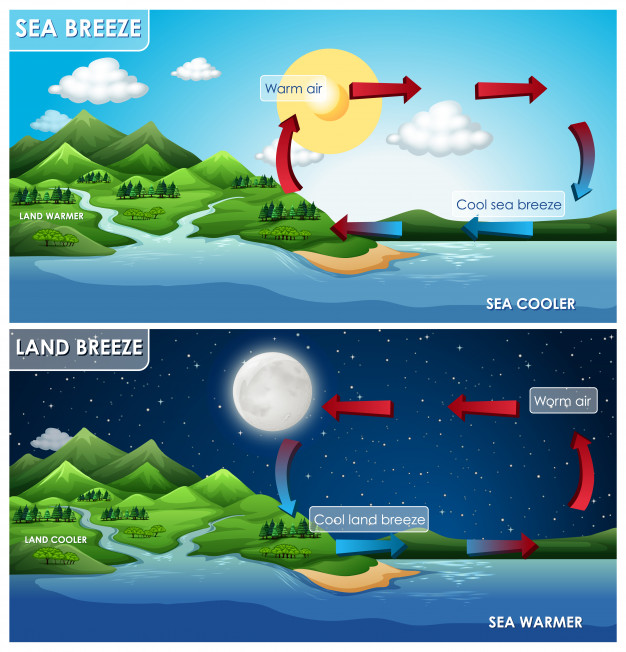• SECTION 01

(a)(i)Bimetallic strip

Temperature sensitive metallic strip which contain two metal rods with different thermal expansivity riveted together. When a sufficient heat is applied to a bimetallic strip, the strip bend corresponding to amount of heat applied on it.

(ii)Linear expansivity of the solid

Linear expansivity of the solid is the fractional increase in length of a solid object, per unit rise in temperature. Linear expansivity is measured in change in length (∆L) per unit raise in temperature (℃)

• SECTION 02

(a)(i)In cold weather the metal blade of a knife feels cooler than the wooden handle

The key point to this phenomenon is high thermal conductivity of metals which cause rate at which heat flow from hand to the metal to be relatively faster compared to that of the wood.

(ii)A cool breeze blows from the sea on a hot summer dayDuring day time sun radiation heat both land and ocean water however due to high heat capacity of water compared to heat capacity of the land, sea water become relatively cool together with air above sea on the other side the land becomes warmer together with the air on the land. Since warm air expands, its density becomes less and consequently atmospheric pressure decreases on land side but on the sea side cool water results on cool air with high density hence the atmospheric pressure increases. Difference in atmospheric pressure between ocean and land cause a cool breeze to flow from sea (on high atmospheric pressure) to land (on low atmospheric pressure).

• SECTION 03

(c)(i)Latent heat of fusion

Latent heat of fusion is the change in enthalpy based on the provided energy, typically heat, to a specific quantity of the substance to change its state from a solid to a liquid, at constant pressure.

(ii) (ii) Copper block of mass 0.68kg is suspended in a freezing mixture at -50C for some time and then transferred to a large volume of water at 0C. Calculate the mass of ice formed.

Data & Reasoning:

Mass of Copper = 0.68kg

Initial temperature of Copper = -50

Specific Heat Capacity (SHC) of Copper = 385 J/Kg

Latent heat of fusion of water (LHF) = 334,800J/Kg

Absorbed thermal energy from water to copper, will be equivalent to Amount of ice generated

Absorbed thermal energy = Specific Heat Capacity X Mass X Change in Temperature

Solidification energy = Latent heat X Mass of solid

Specific Heat Capacity X Mass X Change in Temperature = Latent heat X Mass of solid

385 J/Kg x 0.68Kg x (0 – (-50)) = 334,800J/Kg x Mass of ice

Mass of ice = (385 J/Kg x 0.68Kg x (0  (-50))℃)/ (334,800J/Kg)  = 0.0391 Kg

Mass of ice = 0.0396 Kg = 39.1 g